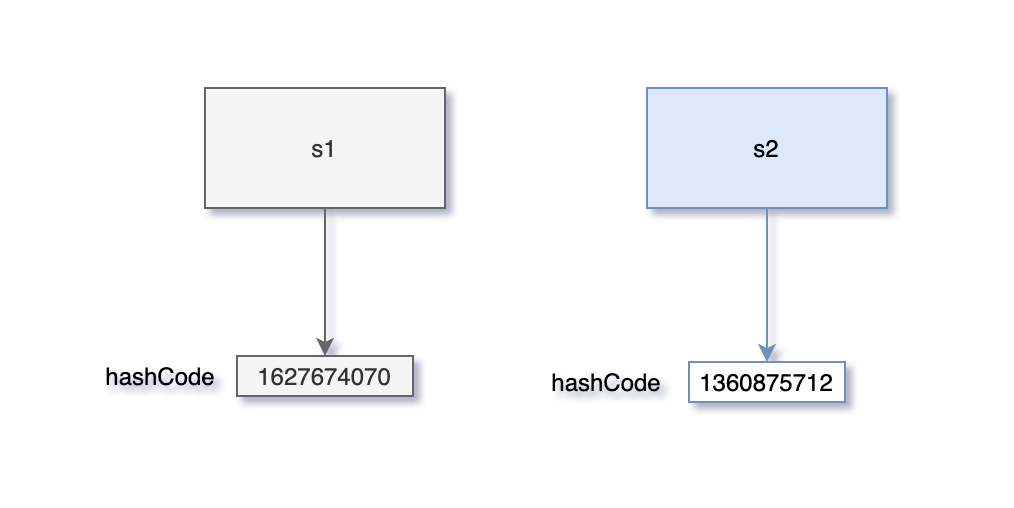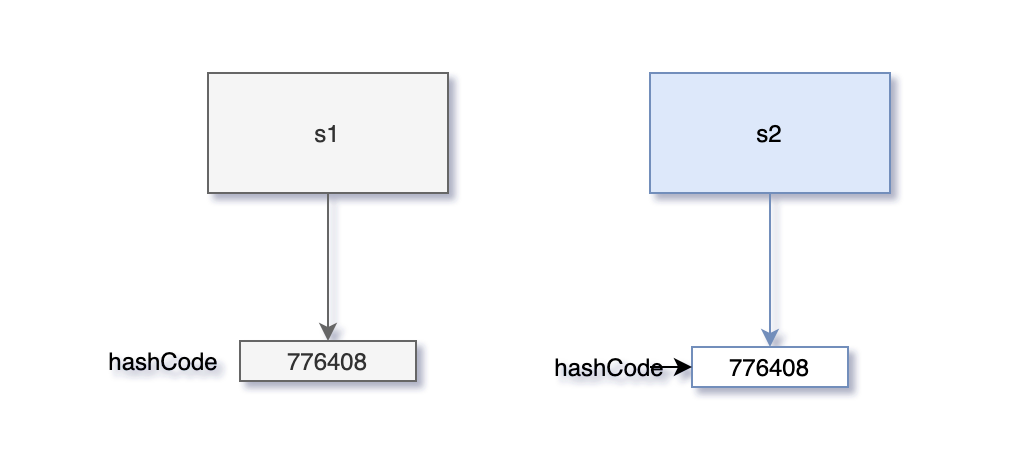# 为什么重写equals方法的时候必须要重写hashCode方法？

2021年10月22日

“二哥，我在读《Effective Java》 的时候，第 11 条规约说重写 equals 的时候必须要重写 hashCode 方法，这是为什么呀？”三妹单刀直入地问。

“三妹啊，这个问题问得非常好，因为它也是面试中经常考的一个知识点。今天哥就带你来梳理一下。”我说。

Java 是一门面向对象的编程语言，所有的类都会默认继承自 Object 类，而 Object 的中文意思就是“对象”。

Object 类中有这么两个方法：

``````public native int hashCode();

public boolean equals(Object obj) {
return (this == obj);
}
``````

1）hashCode 方法

2）equals 方法

“二哥，看起来两个方法之间没有任何关联啊？”三妹质疑道。

“单从这两段解释上来看，的确是这样的。”我解释道，“但两个方法的 doc 文档中还有这样两条信息。”

“哦，这样讲的话，两个方法确实关联上了，但究竟是为什么呢？”三妹抛出了终极一问。

“hashCode 方法的作用是用来获取哈希值，而该哈希值的作用是用来确定对象在哈希表中的索引位置。”我说。

``````public V get(Object key) {
HashMap.Node<K,V> e;
return (e = getNode(hash(key), key)) == null ? null : e.value;
}
``````

``````final HashMap.Node<K,V> getNode(int hash, Object key) {
HashMap.Node<K,V>[] tab; HashMap.Node<K,V> first, e; int n; K k;
if ((tab = table) != null && (n = tab.length) > 0 &&
(first = tab[(n - 1) & hash]) != null) {
if (first.hash == hash && // always check first node
((k = first.key) == key || (key != null && key.equals(k))))
return first;
if ((e = first.next) != null) {
if (first instanceof HashMap.TreeNode)
return ((HashMap.TreeNode<K,V>)first).getTreeNode(hash, key);
do {
if (e.hash == hash &&
((k = e.key) == key || (key != null && key.equals(k))))
return e;
} while ((e = e.next) != null);
}
}
return null;
}
``````

HashMap 是通过拉链法来解决哈希冲突的，也就是如果发生哈希冲突，同一个键的坑位会放好多个值，超过 8 个值后改为红黑树，为了提高查询的效率。

“O(n) 和 O(1) 是什么呀？”三妹有些不解。

“这是时间复杂度的一种表示方法，随后二哥专门给你讲一下。简单说一下 n 和 1 的意思，很显然，n 和 1 都代表的是代码执行的次数，假如数据规模为 n，n 就代表需要执行 n 次，1 就代表只需要执行一次。”我解释道。

“三妹，你想一下，如果没有哈希表，但又需要这样一个数据结构，它里面存放的数据是不允许重复的，该怎么办呢？”我问。

“要不使用 equals 方法进行逐个比较？”三妹有些不太确定。

“这种方法当然是可行的，就像 `if ((e = first.next) != null) {}` 子句中那样，但如果数据量特别特别大，性能就会很差，最好的解决方案还是 HashMap。”

HashMap 本质上是通过数组实现的，当我们要从 HashMap 中获取某个值时，实际上是要获取数组中某个位置的元素，而位置是通过键来确定的。

``````public V put(K key, V value) {
return putVal(hash(key), key, value, false, true);
}
``````

``````final V putVal(int hash, K key, V value, boolean onlyIfAbsent,
boolean evict) {
HashMap.Node<K,V>[] tab; HashMap.Node<K,V> p; int n, i;
if ((tab = table) == null || (n = tab.length) == 0)
n = (tab = resize()).length;
if ((p = tab[i = (n - 1) & hash]) == null)
tab[i] = newNode(hash, key, value, null);
else {
// 拉链
}
return null;
}
``````

``````static final int hash(Object key) {
int h;
return (key == null) ? 0 : (h = key.hashCode()) ^ (h >>> 16);
}
``````

“那二哥，你好像还是没有说为什么重写 equals 方法的时候要重写 hashCode 方法呀？”三妹忍不住了。

“来看下面这段代码。”我说。

``````public class Test {
public static void main(String[] args) {
Student s1 = new Student(18, "张三");
Map<Student, Integer> scores = new HashMap<>();
scores.put(s1, 98);

Student s2 = new Student(18, "张三");
System.out.println(scores.get(s2));
}
}
class Student {
private int age;
private String name;

public Student(int age, String name) {
this.age = age;
this.name = name;
}

@Override
public boolean equals(Object o) {
Student student = (Student) o;
return age == student.age &&
Objects.equals(name, student.name);
}
}
``````

``````null
``````

“二哥，怎么输出了 null，而不是预期当中的 98 呢？”三妹感到很不可思议。

“原因就在于重写 equals 方法的时候没有重写 hashCode 方法。”我回答道，“equals 方法虽然认定名字和年纪相同就是同一个学生，但它们本质上是两个对象，hashCode 并不相同。”“那怎么重写 hashCode 方法呢？”三妹问。

“可以直接调用 Objects 类的 hash 方法。”我回答。

`````` @Override
public int hashCode() {
return Objects.hash(age, name);
}
``````

Objects 类的 hash 方法可以针对不同数量的参数生成新的哈希值，hash 方法调用的是 Arrays 类的 hashCode 方法，该方法源码如下：

``````public static int hashCode(Object a[]) {
if (a == null)
return 0;

int result = 1;

for (Object element : a)
result = 31 * result + (element == null ? 0 : element.hashCode());

return result;
}
``````

``````result = 31*1 + Integer(18).hashCode();
``````

``````result = (31*1 + Integer(18).hashCode()) * 31 + String("张三").hashCode();
``````

``````98
``````“每当重写 equals 方法时，hashCode 方法也需要重写，原因就是为了保证：如果两个对象调用 equals 方法返回的结果为 true，那么两个对象调用 hashCode 方法返回的结果也必然相同。”我点题了。

“OK，get 了。”三妹开心地点了点头，看得出来，今天学到了不少。